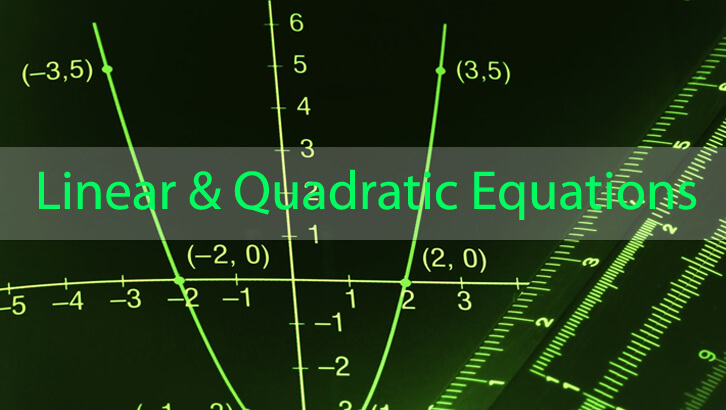# Interesting question from yahoogroups

This one was posted on our yahoogroups. The discussion was also interesting. This is one of my favourite questions. Probably the best question that has been posted to the groups this past month. Just thought I would capture the essence of the mail thread on this post.

Question

Let the cost of 3 apples and 4 oranges be Rs 21. If Anjli can buy at most 4 apples and 3 oranges with Rs 20 and no more fruit, then the maximum amount that could be left with Anjli will be about?

First set of replies

· Ans is 2.50 /- . correct me if i am wrong

· If each apple cost 33paisa and oranges 5 rupees then Anjali can buy 3 apples and 4 apples for 21then 4 apples and 3 oranges will cost 16.2 which means that she can have 3.8 rs. For such problems u need to assign maximum values to more quantities and then should proceed.

· say let me follow with yo approach! even then ! i can assume value 5.1 for b? right ? and 4*(5.1) + 3*(0.2) = 21. so here b = 5.1 and a=0.2 and 4(0.2) + 3(5.1) will be 16.1 and the answer would be 3.9 right ?? Kindly any one reply to this !

· Orange-Rs. 4, Apple-Rs. 1.66. Balance- Rs. 1.33, Hope You got it

Question recap (this mail sent by yours truly, must confess that I fell for the trap)

Let the cost of 3 apples and 4 oranges be Rs 21. If Anjli can buy at most 4 apples and 3 oranges with Rs 20 and no more fruit, then the maximum amount that could be left with Anjli will be about?

I would go for pricing apples at 0, price per orange would be Rs. 5.25 Cost of 4 apples and 3 oranges = 3* 5.25 + 0 = Rs.15.75, which leaves us with Rs. 4.25

Of course, this assumes that the price of apple cannot be negative 🙂

Correct Solution – This is a beautiful solution.

all are missing one point that with 20 she can not buy any more fruit ..means the money left is less than price (minimum) of any og the two friuts…otherwise she can buy more friuts, so max amount of money left can be just higher than cost of chaper fruit.

Now 4x+3y =21

also 3x+4y+left money =20

3x+4y+y=20(leftmoney=cost of cheaper fruit, y will be chaper as on exchange of qunatity total value decreases or 4x+3y>3x+4y……….x>y)

4x+3y =21

3x+5y =20

solving this y=17/11=1.545 which is the answer

This is why this is a beautiful question (final reply from question-provider)

@sony, thank you so much, thats exactly what we were missing

a)1b)1.25c)2d)2.25e)1.5

these are the options given,

from the options given i was ignoring the costs that include denominations other than normally what we see, but i’m getting the answer as .75(with apple=2 and orange=3.75)

from your solution the answer is 1.5, i guess thats the correct one from the options.

Posted by

Rajesh B Director, 2IIM

CAT correspondence and classroom coaching @ Chennai, CAT Online Coaching